## New Maths Curriculum: Year 2 Fractions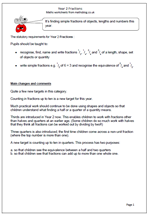Fractions are seen as a completely separate area now, rather than being within Number or Division and Fractions.

The statutory requirements for Year 2 Fractions :

Pupils should be taught to:

•    recognise, find, name and write fractions 1/3, 1/4, 2/4 and 3/4 of a length, shape, set of objects or quantity

•    write simple fractions e.g. 1/2 of 6 = 3 and recognise the equivalence of 2/4 and 1/2.

Quite a few new targets in this category.
Counting in fractions up to ten is a new target for this year.
Much practical work should continue to be done using shapes and objects so that children understand what finding a half or a quarter of a quantity means.

Thirds are introduced in Year 2 now. This enables children to work with fractions other than halves and quarters at an earlier age. (Some children do so much work with halves that they think all fractions can be worked out by dividing by two!!)

Three quarters is also introduced; the first time children come across a non-unit fraction (where the top number is more than one).

A new target is counting up to ten in quarters. This process has two purposes:

a. so that children see the equivalence between a half and two quarters
b. so that children see that fractions can add up to more than one whole one.

New Maths Curriculum: Fractions

## New Maths Curriculum: Year 1 Fractions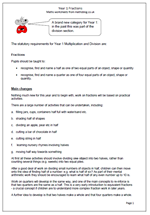Fractions is a brand new category for Year 1: in the past this was part of the division section.

The statutory requirements for Year 1 Multiplication and Division are:

Fractions

Pupils should be taught to:

•    recognise, find and name a half as one of two equal parts of an object, shape or quantity

•    recognise, find and name a quarter as one of four equal parts of an object, shape or quantity.

Main changes

Nothing much new for this year and to begin with, work on fractions will be based on practical activities.

There are a large number of activities that can be undertaken, including:

a.    filling jars, cups, containers half full with water/sand etc.

c.    dividing an apple, pear etc in half

d.    cutting a bar of chocolate in half

e.    cutting string in half

f.     learning nursery rhymes involving halves

g.    moving half way towards something

At first all these activities should involve dividing one object into two halves, rather than counting several things (e.g. sweets) into two equal piles.

After a good deal of work on dividing small numbers of objects in half, children can then move onto the idea of finding half of a number: e.g. what is half of six? As part of their mental arithmetic work they should be encouraged to learn what half of any even number up to 10 is.

Work on quarters will develop in the same way, and one of the main concepts to re-inforce is that two quarters are the same as a half. This is a very early introduction to equivalent fractions – a crucial concept if children are to understand more complex fraction work in later years.

A further idea to develop is that two halves make a whole and that four quarters make a whole.

Year 1 Fractions: statutory requirements

## Resource of the Week: Find tenths and hundredths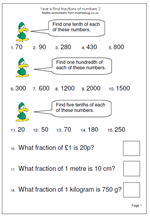This week we take a look at a worksheet on the relationship between fractions and division. It is important that children understand that finding one half of a number is equivalent to dividing by 2, that finding one tenth of a number is the same as dividing the number by 10 and to find one hundredth is equivalent to dividing by 100. Plenty of practice is needed with this before children go on to find, for example, two tenths, or three hundredths of numbers.

Most of these questions should be calculated mentally, although rough jottings may help. The second set of questions could cause a few problems. For example: ‘What fraction of a £1 is 20 ?’ requires a couple of steps:

Firstly convert the pound to pence £1 = 100p

Secondly write the fraction as 20 out of 100 or 20/100

Thirdly simplify to 2/10 and then 1/5.

Find fractions of numbers (p2)

## More on equivalent fractions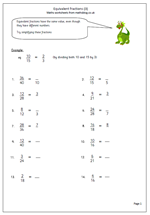Equivalent fractions are fractions which look different but have the same value. The easiest example of equivalent fractions is a half and two quarters. If I have half of a fruit cake that is the same as having two quarters of the same cake. (As long as I pick up all the crumbs from cutting it!)

Not all equivalent fractions are that easy to work out, but as a rule it can be said that:

If we multiply the top and bottom numbers in a fraction by the same value we will get an equivalent fraction.

The same can be said of division:

If we divide the top and bottom numbers of a fraction by the same value we will get an equivalent fraction.

Dividing is really useful as it ‘simplifies’ the fraction, often making it easier to read or to picture. For example 10/100 is equivalent to 1/10 as both top and bottom have been divided by 10.

Now, the fraction will only get simpler if we can successfully divide both top and bottom numbers without leaving a remainder! This is the key, and, as so often, a good knowledge of tables helps here.

This worksheet looks at simplifying fractions. As shown above, this is done by dividing the top and bottom of the fraction by the same number. This division process might need to be done more than once. For example: 8/12 can be simplified to 4/6. This in turn can be simplified to 2/3.

Equivalent fractions (pg 3)

## Year 6 maths worksheet: Adding fractions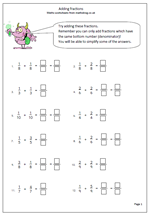One of the big stumbling blocks in maths is working with fractions, especially addition, subtraction, multiplication and division. The problem is caused by a lack of understanding of what a fraction actually means and how they can be manipulated to make the task easier. (Equivalent fractions being the key.)

A common fault when adding fractions is for a child to simply add the two top numbers and then add the two bottom numbers. This will always lead to a wrong answer and it is important to stress to children that to add two fractions the bottom numbers (denominators) must be the same. The reason for this can easily be shown by cutting a shape in to different sized parts and then asking how much two of the parts could be.

This particular worksheet takes all the stress out of the problem as the denominators are already the same for each addition question. Where an answer can be simplified a further answer is expected.

## Year 6 Equivalent Fractions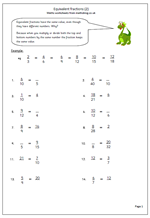Here is another page of equivalent fractions. One part of each pair of fractions is missing and the missing numerator or denominator needs to be entered. Remember, equivalent fractions are fractions which have the same overall value.

One interesting way to check whether two fractions are equivalent is to carry out a couple of multiplications; what is known as cross multiplication. To check whether a/b is equivalent to c/d just multiply a by d and then multiply b by c. If the two answers are equal then the fractions are equivalent. This is a really good way to check to see whether the answers given are correct.

Equivalent fractions (pg 2)

## Year 6 Maths Worksheet: Equivalent fractions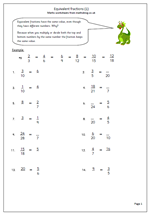Understanding equivalent fractions is at the heart of understanding how fractions work. Much work needs to be done in earlier years to show that fractions such as 1/2 and 2/4 have the same value. This is usually done by colouring or shading parts of rectangles, circles.

Two fractions which are equal in value we say are equivalent.

2/5 is equivalent to 4/10 which is equivalent to 40/100.

If we start with a fraction and multiply both the numerator (top number) and the denominator (bottom number) by the same number the fraction will remain with the same value. The same is true with division, but not addition or subtraction.

This makes multiplying fractions much easier than adding or subtracting them.

Equivalent fractions (pg 1)

## Year 6 maths worksheet: adding fractions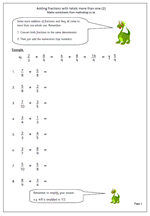My first addition of fractions page was very popular and I have had  several requests for more, as it does take some time and re-inforcement to get the hang of this concept. I believe that adding fractions is one of the hardest topics for children to understand, mainly because it involves so many steps.

Remember, to add two fractions the denominator has to be the same: this requires a good understand of equivalent fractions, so make sure that this is fully understood before attempting addition. Once this has been done the addition is easy, as the denominator stays the same and just the top numbers (numerators) are added.

This will result in a vulgar fraction eg 14/10 which needs to be converted to a mixed number.

As there are ten tenths in one whole one this makes 1 and 4/10.

Finaly the fraction can be simplified: 4/10 is the same as 2/5 so the final answer will be 1 and 2/5.

Adding fractions that total more than 1 (pg 2)

## Year 5 ordering fractions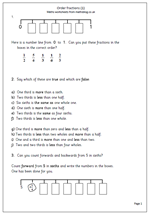Fractions always seem to cause problems for children who are less confident with their maths, often because they do not get enough practice with ordering and comparing fractions. This worksheet compares sixths and thirds by placing them in order on a number line as well as asking whether statements are true or false. A good understanding of equivalent fractions is needed to do this and many children will still need practice at counting forwards and backwards in fractions (eg count on from zero in sixths up to 2 whole ones.)

Order fractions (1)

## Year 4 maths worksheets on fractions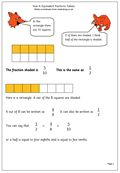Fractions form an important part of the maths for Year 4 children (aged 8/9) and often it turns out to be one of the hardest to get to grips with.

One important aspect of this is to identify equivalent fractions (in other words, that a half is the same as two quarters or four tenths is the same as two fifths etc.) This is usually introduced by using diagrams, but many children fail to see beyond the colouring of a diagram to the more abstract idea of equivalence.

Children will also work with mixed numbers, placing them on number lines and to identify pairs of fractions which total 1.

Another important skill is to recognise the equivalence between the decimals and fractions for simple fractions such as: 0.5 =a half, 0.25 = a quarter, 0.1 = a tenth and 0.01 = a hundredth.

I have published a number of worksheets to help with this and coverage is increasing steadily. Already there are pages on equivalent fractions, tenths, ordering fractions and much more. These can be found in the year 4 Counting and Number section of the site.

Go to our year 4 maths worksheets on fractions